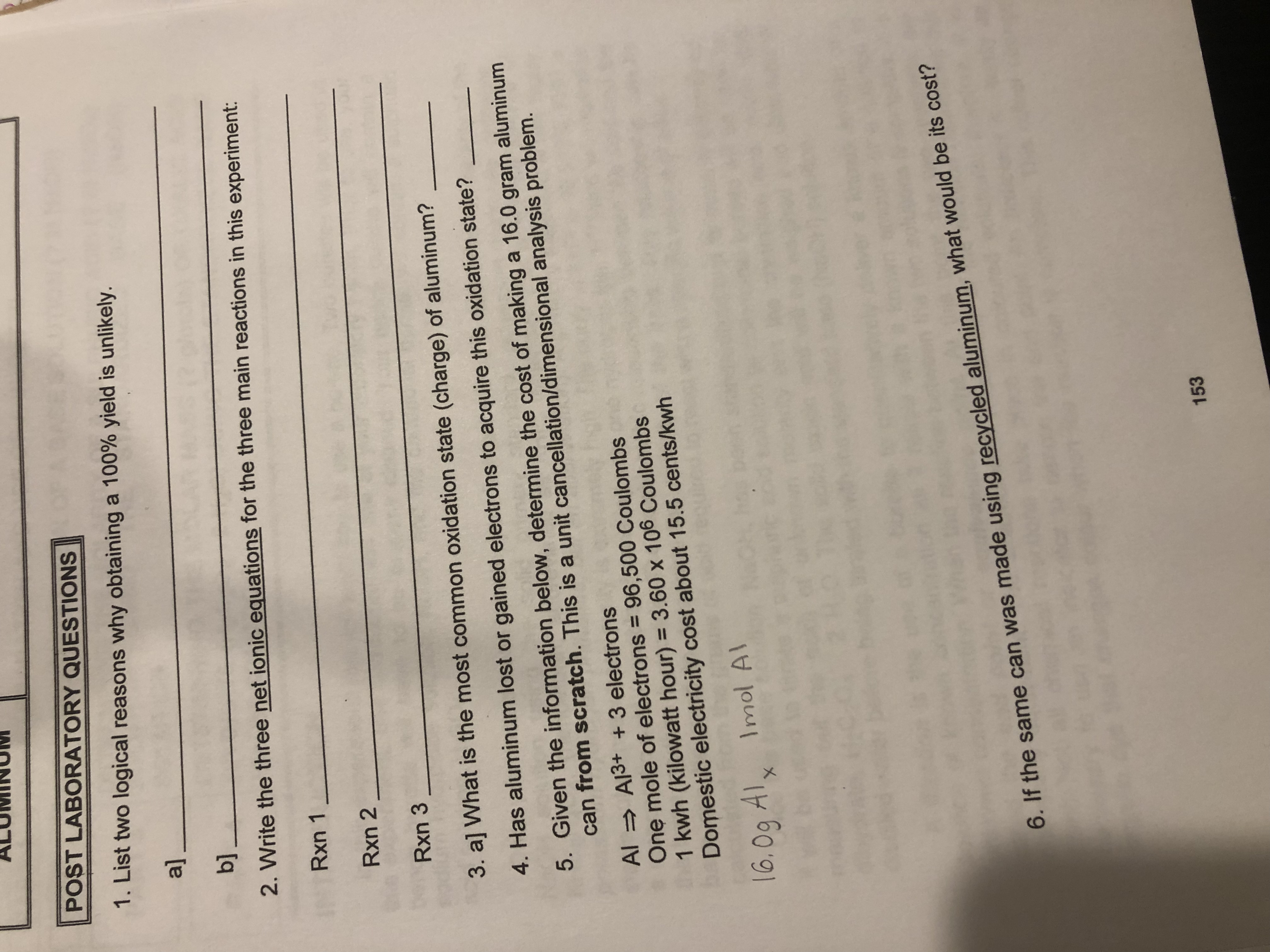# POST LABORATORY QUESTIONS1. List two logical reasons why obtaining a 100% yield is unlikely.alb]_2. Write the three net ionic equations for the three main reactions in this experiment:Rxn 1Rxn 2Rxn 33. a] What is the most common oxidation state (charge) of aluminum?4. Has aluminum lost or gained electrons to acquire this oxidation state?5. Given the information below, determine the cost of making a 16.0 gram aluminumcan from scratch. This is a unit cancellation/dimensional analysis problem.Al Al3+ 3 electronsOne mole of electrons 96,500 Coulombs1 kwh (kilowatt hour) 3.60 x 106 CoulombsDomestic electricity cost about 15.5 cents/kwh16.0g AlxImol Al6. If the same can was made using recycled aluminum, what would be its cost?153

Question
6 views

Questions 5help_outlineImage TranscriptionclosePOST LABORATORY QUESTIONS 1. List two logical reasons why obtaining a 100% yield is unlikely. al b]_ 2. Write the three net ionic equations for the three main reactions in this experiment: Rxn 1 Rxn 2 Rxn 3 3. a] What is the most common oxidation state (charge) of aluminum? 4. Has aluminum lost or gained electrons to acquire this oxidation state? 5. Given the information below, determine the cost of making a 16.0 gram aluminum can from scratch. This is a unit cancellation/dimensional analysis problem. Al Al3+ 3 electrons One mole of electrons 96,500 Coulombs 1 kwh (kilowatt hour) 3.60 x 106 Coulombs Domestic electricity cost about 15.5 cents/kwh 16.0g Alx Imol Al 6. If the same can was made using recycled aluminum, what would be its cost? 153 fullscreen
check_circle

Step 1

In any reaction the actual yield is mostly less than theore...

### Want to see the full answer?

See Solution

#### Want to see this answer and more?

Solutions are written by subject experts who are available 24/7. Questions are typically answered within 1 hour.*

See Solution
*Response times may vary by subject and question.
Tagged in

### General Chemistry